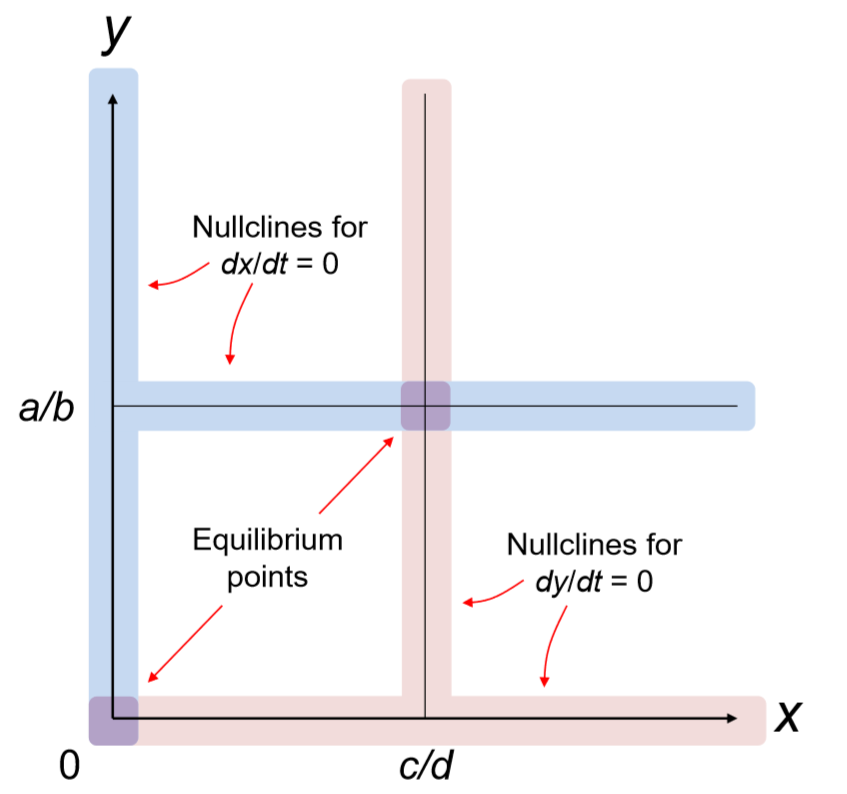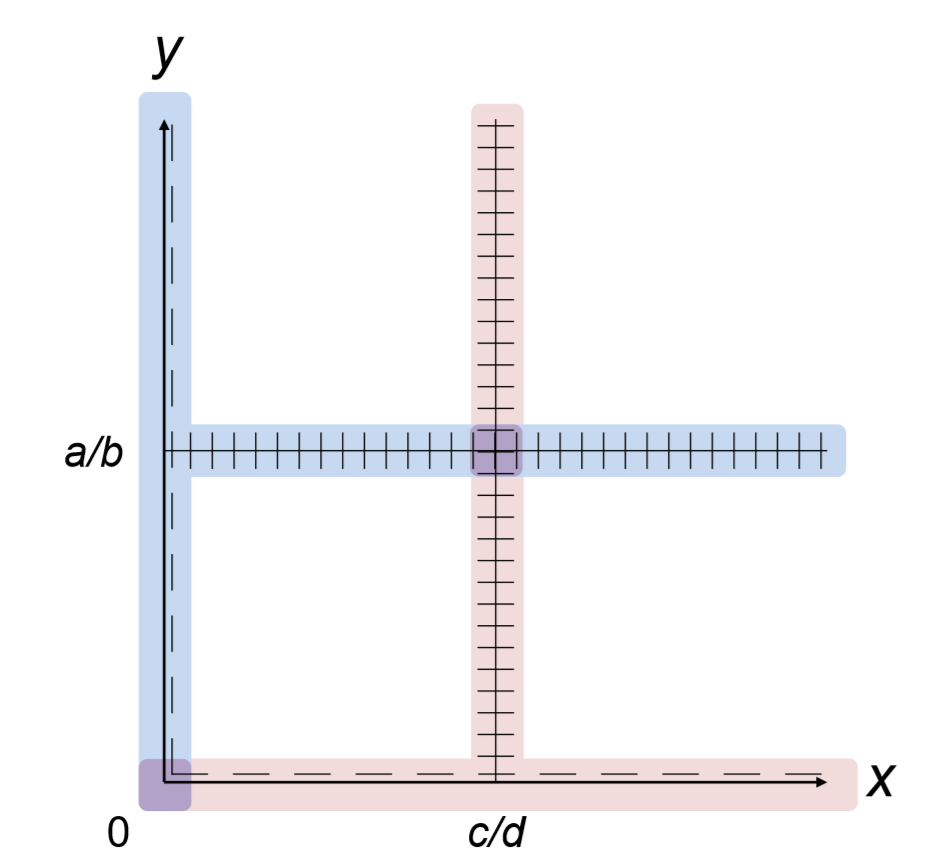Skip to main content
$$\newcommand{\id}{\mathrm{id}}$$ $$\newcommand{\Span}{\mathrm{span}}$$ $$\newcommand{\kernel}{\mathrm{null}\,}$$ $$\newcommand{\range}{\mathrm{range}\,}$$ $$\newcommand{\RealPart}{\mathrm{Re}}$$ $$\newcommand{\ImaginaryPart}{\mathrm{Im}}$$ $$\newcommand{\Argument}{\mathrm{Arg}}$$ $$\newcommand{\norm}{\| #1 \|}$$ $$\newcommand{\inner}{\langle #1, #2 \rangle}$$ $$\newcommand{\Span}{\mathrm{span}}$$

# 7.2: Phase Space Visualization

$$\newcommand{\vecs}{\overset { \rightharpoonup} {\mathbf{#1}} }$$

$$\newcommand{\vecd}{\overset{-\!-\!\rightharpoonup}{\vphantom{a}\smash {#1}}}$$

A phase space of a continuous-time model, once time is discretized, can be visualized in the exact same way as it was in Chapter 5, using Codes 5.1 or 5.2. This is perfectly ﬁne. In the meantime, Python’s matplotlib has a specialized function called streamplot, which is precisely designed for drawing phase spaces of continuous-time models. It works only for two-dimensional phase space visualizations, but its output is quite neat and sophisticated. Here is how you can use this function:The streamplot function takes four arguments. The ﬁrst two (xvalues and yvalues) are discretized x-and y-values in a phase space, each of which is given as a two-dimensional array. The meshgrid function generates such array’s for this purpose. The 0.1 in arange determines the resolution of the space. The last two arguments (xdot and ydot) describe the values of $$dx/dt$$ and $$dy/dt$$ on each point. Each of xdot and ydot should also be given as a two-dimensional array, but since xvalues and yvalues are already in the array structure, you can conduct arithmetic operations directly on them, as shown in the code above. In this case, the model being visualized is the following simple predator-prey equations:

$\frac{dx}{dt} =x-xy \label{(7.10)}$

$\dfrac{dy}{dt} =-y +xy \label{(7.11)}$

The result is shown in Fig. 7.2.1. As you can see, the streamplot function automatically adjusts the density of the sample curves to be drawn so that the phase space structure is easily visible to the eye. It also adds arrow heads to the curves so we can understand which way the system’s state is ﬂowing.

One nice feature of a continuous-time model’s phase space is that, since the model is described in continuous differential equations, their trajectories in the phase space are all smooth with no abrupt jump or intersections between each other. This makes their phase space structure generally more visible and understandable than those of discrete-time models.

Exercise $$\PageIndex{1}$$

Draw a phase space of the following differential equation (motion of a simple pendulum) in Python:

$\frac{d^{2}\theta}{dt^{2}} =-\frac{g}{L}\sin\theta\label{(7.12)}$

Moreover, such smoothness of continuous-time models allows us to analytically visualize and examine the structure of their phase space. A typical starting point to do so is to ﬁnd the nullclines in a phase space. A nullcline is a set of points where at least one of the time derivatives of the state variables becomes zero. These nullclines serveFigure $$\PageIndex{1}$$: Phase space drawn with Code 7.1.

as “walls” that separate the phase space into multiple contiguous regions. Inside each region, the signs of the time derivatives never change (if they did, they would be caught in a nullcline), so just sampling one point in each region gives you a rough picture of how the phase space looks. Let’s learn how this analytical process works with the following $$Lotka-Volterra model$$:

$\frac{dx}{dt} =ax -bxy \label{(7.13)}$

$\frac{dy}{dt} =-cy +dxy\label{(7.14)}$

$x \geq0, y\geq0, a>0, b>0, c>0, d>0 \label{(7.15)}$

First, ﬁnd the nullclines. This is a two-dimensional system with two , so there must be two sets of nullclines; one set is derived from $$\frac{dx}{dt} = 0$$, and another set is derived from $$\frac{dy}{dt} = 0$$. They can be obtained by solving each of the following equations:

$0=ax -bxy \label{(7.16)}$

$0=-cy+dxy \label{(7.17)}$

The ﬁrst equation gives

$x=0, or \ y =\frac{a}{b}. \label{(7.18)}$

These are two straight lines, which constitute one set of nullclines for \frac{dx}{dt} = 0\) (i.e., you could call each line a single nullcline). In the meantime, the second one gives

$y=0, or \ x =\frac{c}{d}. \label{(7.19)}$

Again, these two lines constitute another set of nullclines for $$\frac{dy}{dt} = 0$$. These results can be visualized manually as shown in Fig. 7.2.2. Equilibrium points exist where the two sets of nullclines intersect.Figure $$\PageIndex{2}$$: Drawing a phase space (1): Adding nullclines.

Everywhere on the ﬁrst set of nullclines, $$\frac{dx}{dt}$$ is zero, i.e., there is no “horizontal” movement in the system’s state. This means that all local trajectories on and near those nullclines must be ﬂowing vertically. Similarly, everywhere on the second set of nullclines, $$\frac{dy}{dt}$$ is zero, therefore there is no “vertical” movement and all the local trajectories ﬂow horizontally. These facts can be indicated in the phase space by adding tiny line segments onto each nullcline (Fig. 7.2.3).Figure $$\PageIndex{3}$$: Drawing a phase space (2): Adding directions to the nullclines.

Now the phase space is divided into four regions. It is guaranteed that the trajectories in each of those regions ﬂow only in one of the following four directional categories:

$\cdot \frac{dx}{dt} >0, \frac{dy}{dt} >0("to Northeast")$

$\cdot\frac{dx}{dt} <0, \frac{dy}{dt} > (to"Northwest")$

$\cdot\frac{dx}{dt} >0, \frac{dy}{dt} <0 (to "Southeast")$

$\cdot\frac{dx}{dt} <, \frac{dy}{dt} <0(to"Southwest")$

Inside any region, a trajectory never switches between these four categories, because if it did, such a switching point would have to have already appeared as part of the nullclines. Therefore, sampling just one point from each region is sufﬁcient to know which direction the trajectories are ﬂowing in. For example, you can pick $$(2c/d,2a/b)$$ as a sample point in the upper right region. You plug this coordinate into the model equations to obtain

$\frac{dx}{dt}|_{(x,y) =(\frac{2c}{d}, \frac{2a}{b})}=a\frac{2c}{d}-b\frac{2c}{d}\frac{2a}{b} =-\frac{2ac}{d} <0,\label{(7.20)}$

$\frac{dy}{dt}|_{(x,y)= (\frac{2c}{d},\frac{2a}{b})}=-c\frac{2a}{b} +d\frac{2c}{d}\frac{2a}{b}=\frac{2ac}{b}>-.\label{(7.21)}$

Therefore, you can tell that the trajectories are ﬂowing to “Northwest” in that region. If you repeat the same testing for the three other regions, you obtain an outline of the phase space of the model shown in Fig. 7.2.4, which shows a cyclic behavior caused by the interaction between prey ($$x$$) and predator ($$y$$) populations.Figure $$\PageIndex{4}$$: Drawing a phase space (3): Adding directions of trajectories in each region.

This kind of manual reconstruction of phase space structure can’t tell you the exact shape of a particular trajectory, which are typically obtained through numerical simulation. For example, in the phase space manually drawn above, all we know is that the system’s behavior is probably rotating around the equilibrium point at $$(x,y)$$ = $$(c/d,a/b)$$, but we can’t tell if the trajectories are closed orbits, spiral into the equilibrium point, or spiral away from the equilibrium point, until we numerically simulate the system’s behavior.

Having said that, there is still merit in this analytical work. First, analytical calculations of nullclines and directions of trajectories provide information about the underlying structure of the phase space, which is sometimes unclear in a numerically visualized phase space. Second, analytical work may allow you to construct a phase space without specifying detailed parameter values (as we did in the example above), whose result is more general with broader applicability to real-world systems than a phase space visualization with speciﬁc parameter values.

Exercise $$\PageIndex{1}$$

Draw an outline of the phase space of the following SIR model (variable $$R$$ is omitted here) by studying nullclines and estimating the directions of trajectories within each region separated by those nullclines.

$\frac{dS}{dt} =-aSI \label{(7.22)}$

$\frac{dI}{dt} =aSI-bI\label{(7.23)}$

$S\geq 0, I\geq 0, a>0, b>0\label{(7.24)}$

Exercise $$\PageIndex{2}$$

Draw an outline of the phase space of the following equation by studying nullclines and estimating the directions of trajectories within each region separated by those nullclines.

$\frac{d^{2}x}{dt^{2}}-x\frac{dx}{dt}+x^{2} =0 \label{(7.25)}$